# Test: Variable Separable Form

## 10 Questions MCQ Test Mathematics (Maths) Class 12 | Test: Variable Separable Form

Description
This mock test of Test: Variable Separable Form for JEE helps you for every JEE entrance exam. This contains 10 Multiple Choice Questions for JEE Test: Variable Separable Form (mcq) to study with solutions a complete question bank. The solved questions answers in this Test: Variable Separable Form quiz give you a good mix of easy questions and tough questions. JEE students definitely take this Test: Variable Separable Form exercise for a better result in the exam. You can find other Test: Variable Separable Form extra questions, long questions & short questions for JEE on EduRev as well by searching above.
QUESTION: 1

### Write the order and degree of the given differential equation: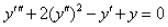​

Solution:

Order = 3 and Degree = 1
The order of a differential equation is determined by the highest-order derivative; the degree is determined by the highest power on a variable. The higher the order of the differential equation, the more arbitrary constants need to be added to the general solution.

QUESTION: 2

### The order of a differential equation representing a family of curves is same as:​

Solution:

The order of a differential equation representing a family of curves is same as the number of arbitrary constants present in the equation corresponding to the family of curves.

QUESTION: 3

### The solution of the equation x3dx + (y + 1)2 dy = 0

Solution:

x3dx + (y + 1)dy = 0
=> d(x4/4) + d((y + 1)3/3) = 0
=> d(x4/4 + ((y + 1)3/3)) = 0
=> (x4/4 + ((y + 1)3/3)) = constt
=> 12 *(x4/4 + ((y + 1)3/3)) = 12 * constt
=> 3x4 + 4(y + 1)3 = constt

QUESTION: 4

Identify the type of differential equation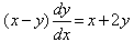Solution:

(x−y)dy/dx = x + 2y
⇒ dy/dx = (x + 2y)/(x−y)
F (x,y) = (x + 2y)/(x−y)
F(Ax, Ay) = (Ax + 2Ay)/(Ax−Ay)
= A(x + 2y)/A(x−y)
= (x + 2y)/(x − y) = F (x,y)
Hence, the equation is homogenous.

QUESTION: 5

Identify the form of the given Differential Equation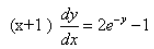Solution:
QUESTION: 6

The solution of the initial value problem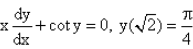is :

Solution:

xdy/dx = -coty
dy/dx = - coty/x
dy/coty = - dx/x
tany dy = - dx/x
∫(siny/cosy)dy = - ∫dx/x…………..(1)
Put t = cosy
dt = -sinydy
Put the value of dt in eq(1)
-∫dt/t = -∫dx/x
log |t| = log |x| + log c
log |cosy| = log(c.|x|)
|cos y| = c.|x|....................(2)
As y = π/4, x = (2)½
cos π/4 = c*(2)½
1/(2)½ = c*(2)½
c = ½
Put the value of ‘c’ in eq(2)
cos y = x/2    => x = 2cosy

QUESTION: 7

The solution of the differential equation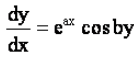is :

Solution:

dy/dx = eax cos by
∫dy/cos by = ∫eax dx
∫sec by dy = ∫eax dx
= (log| sec by + tan by|)/b = eax /a + c
= a(log| sec by + tan by|) = beax + c

QUESTION: 8

The solution of the differential equation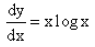is :

Solution:

dy/dx = x logx
=> ∫dy = ∫x logx dx
y = logx . x2/2 - ∫1/x . x2/2 dx + c
y = x2/2 log x -½ ∫x dx + c
y = x2/2 log x - ½ . x2/2 + c
y = x2/2 log x - x2/4 + c

QUESTION: 9

The solution of the differential equation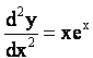is :

Solution:

d2y/dx2 = xex
d2y/dx2 =  ∫xex
d/dx(dy/dx) = [xex]
Integrating, we get
dy/dx = (x-1)ex+ c1
Again integrating, y = (x-2)ex + c1x + c2

QUESTION: 10

The solution of the initial value problem  edy/dx = x + 1, y(0) = 3 is :

Solution:

edy/dx = x + 1
⇒ dy/dx = log(x+1)
⇒ dy = log(x+1)dx
Integrating both sides, we get
⇒ ∫dy =  ∫log(x+1)dx
y = log(x + 1)  ∫1dx - ∫[d/dx{log(x + 1)} ∫1dx]dx
y = xlog(x + 1) -  ∫1/(x+1)xdx
y = xlog(x + 1) -  ∫(1 - 1/(x + 1)dx
y = xlog(x + 1) -  ∫dx +  ∫1/(x+1)dx
y = xlog(x + 1) - x + log|x + 1| + c
y = (x + 1)log|x + 1| - x + c
Here c is 3
y = (x + 1)log|x + 1| - x + 3